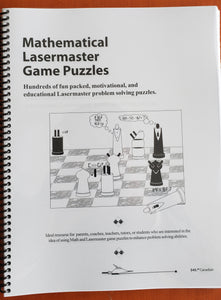Federation of Laser Masters

# Mathematical Laser Master Game Puzzles

Regular price \$30.00 \$0.00

### This book is mandatory for all Laser Master students!

• Addition, subtraction, multiplication and division
• Geometry including shapes, angles, parallel lines, symmetry and solids
• logic of word problems
• statistical tables and charts, data classification
• factors, multiples, fractions, percents and decimals
• Algebraic equations